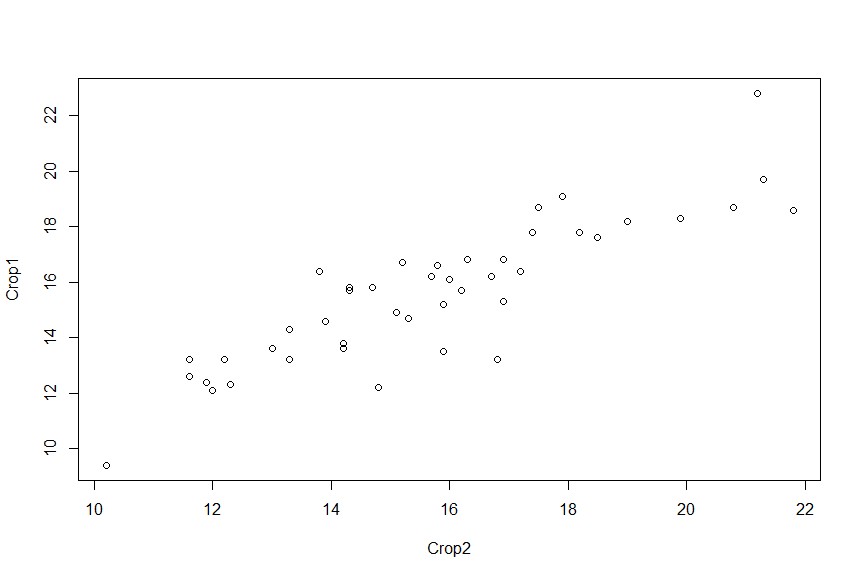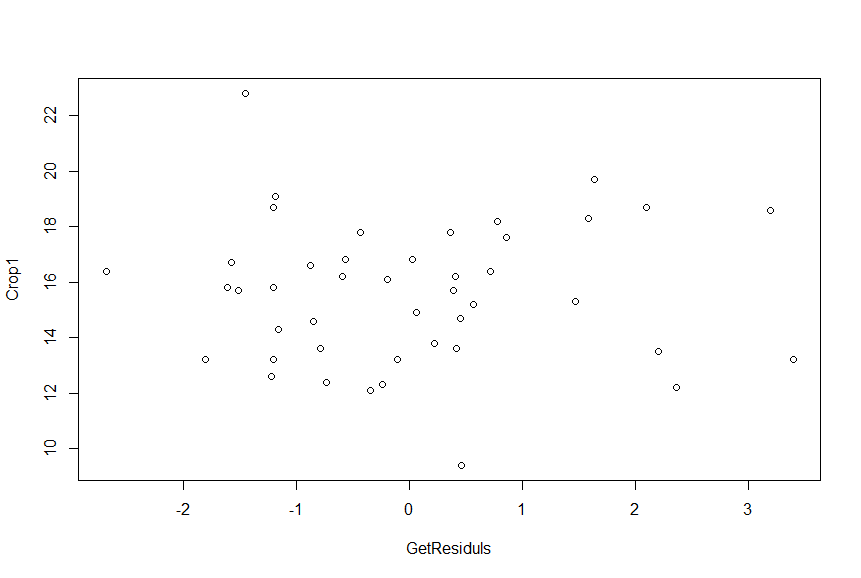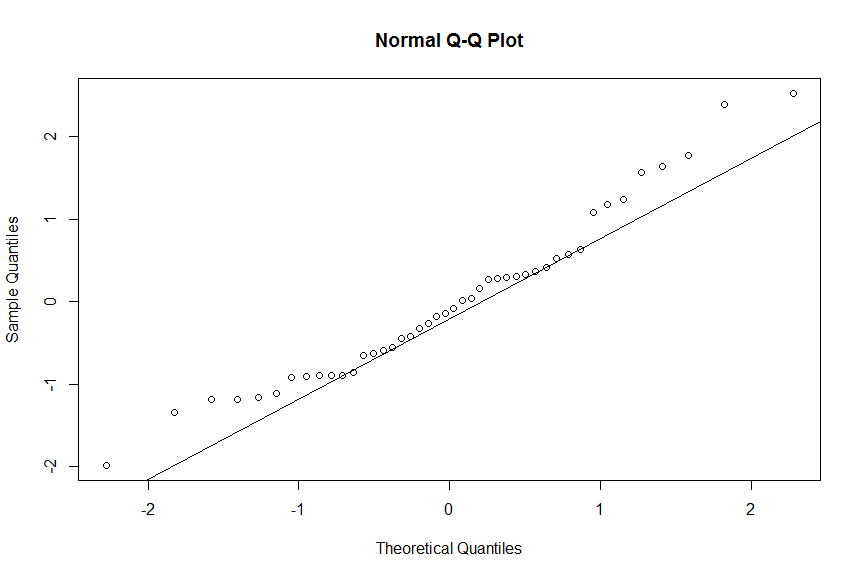# Linear Regression

This document contains the code required to work through the examples given in the Linear Regression segment of module 3. The code is syntax for R, and is given in logical order, so each line can be consecutively copied and pasted into the console in R and the correct plots / data should appear. Detailed explanation on the meaning behind the output of these commands can be found in the pdf in Module 3, Having this pdf file alongside as you work through these commands are highly recommended. Some figures are displayed below to ensure you are obtaining the correct results.

```Crop1 = CropYield[]
Crop2 = CropYield[]
plot(Crop2,Crop1)
Mod1 = lm(Crop2 ~ Crop1, data=CropYield)
summary(Mod1)
confint(Mod1)
bartlett.test(Crop2 ~ Crop1, data = CropYield)
anova(Mod1)
GetResiduls = resid(Mod 1)
plot(GetResiduls, Crop1)
Mod1_studentized = rstandard(Mod1)
qqnorm(Mod1_studentized)
qqline(Mod1_studentized)
```

A plot of Crop2 vs Crop1, or y vs x.This following figure is what should appear after plotting of the residuals with the x values, Crop1.This figure shows the typical Q-Q plot generated after the last line of code above is typed into R. The diagonal line is created from the last command, and the plot iteself is creatted from the QQnorm command.This site uses Akismet to reduce spam. Learn how your comment data is processed.